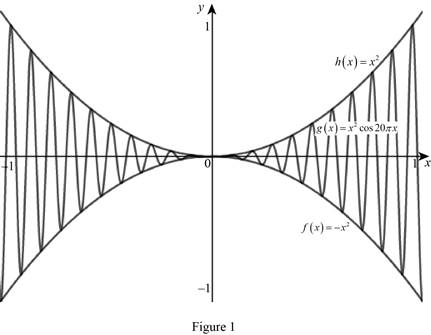# The limit of the function lim x → 0 x 2 cos 20 π x = 0 .### Single Variable Calculus: Concepts...

4th Edition
James Stewart
Publisher: Cengage Learning
ISBN: 9781337687805### Single Variable Calculus: Concepts...

4th Edition
James Stewart
Publisher: Cengage Learning
ISBN: 9781337687805

#### Solutions

Chapter 2.3, Problem 27E
To determine

## To show: The limit of the function limx→0x2cos20πx=0.

Expert Solution

### Explanation of Solution

Theorem used: The Squeeze Theorem

“If f(x)g(x)h(x) when x is near a (except possibly at a) and limxaf(x)=limxah(x)=L then limxag(x)=L.”

Proof:

Apply the Squeeze Theorem and obtain a function f smaller than g(x)=x2cos20πx and a function h bigger than g(x)=x2cos20πx such that both f(x) and h(x) approaches 0.

Since the cosine function is lies between 1 and 1, 1x2cos20πx1.

Any inequality remains true when multiplied by a positive number. Since x20 for all x, multiply each side of the inequalities by x4.

1×x2x2×cos20πx1×x2x2x2cos20πxx2

Let f(x)=x2, g(x)=x2cos20πx and h(x)=x2.

When the limit x approaches zero, the inequality becomes,

limx0(x2)limx0(x2cos20πx)limx0(x2)

Graph:

Sketch the graph of the function by using the online graphing calculator as shown below in Figure (1).From the graph, it is observed that limx0(x2)=0 and limx0(x2)=0.

If f(x)g(x)h(x) when x approaches 0 and limx0(x2)=limx0(x2)=0, then by Squeeze Theorem the limit of the function g(x) is zero.

That is, limx0x2cos20πx=0.

Hence showed.

### Have a homework question?

Subscribe to bartleby learn! Ask subject matter experts 30 homework questions each month. Plus, you’ll have access to millions of step-by-step textbook answers!